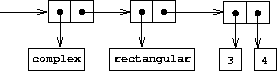# Complex-number selectors

Louis Reasoner tries to evaluate the expression `(magnitude z)` where `z` is the object shown in figure 2.24. To his surprise, instead of the answer `5` he gets an error message from `apply-generic` , saying there is no method for the operation `magnitude` on the types `(complex)` . He shows this interaction to Alyssa P. Hacker, who says ''The problem is that the complex-number selectors were never defined for `complex` numbers, just for `polar` and `rectangular` numbers. All you have to do to make this work is add the following to the `complex` package:''

``````(put 'real-part '(complex) real-part)
(put 'imag-part '(complex) imag-part)
(put 'magnitude '(complex) magnitude)
(put 'angle '(complex) angle)
``````

Describe in detail why this works. As an example, trace through all the procedures called in evaluating the expression `(magnitude z)` where `z` is the object shown in figure 2.24. In particular, how many times is `apply-generic` invoked? What procedure is dispatched to in each case?Figure 2.24: Representation of `3 + 4i` in rectangular form.